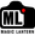# lens.c edited to account for diffraction in depth of field

#632 Merged at 848d753
RepositoryDepth of Field with diffraction
Branch
unified
RepositoryMagic Lantern
Branch
unified
Reviewers
Description

This is my first pull request, so forgive me if I have done it 'wrong'

1.Alex

Actually it's very good, with proper explanation.

The only thing I'd change is indentation (4 spaces) and spacing (to keep it somewhat consistent).

2.Garry George author

Alex

I have looked at your changes and I fear things are now broken.

I think the thing you didn't like with my original coding was the fact that the near and far went to zero.

I did this to flag up that the aperture had gone past an acceptable limit.

Remember we are using a combined blur of defocus and diffraction , combined in quadrature.

It is correct that the acceptable DoF collapses ever closer to the focus point, after it has reached maximum.

This is my tweak of your code: Compute the depth of field, accounting for diffraction. See: http://www.largeformatphotography.info/articles/DoFinDepth.pdf Assumes a ‘generic’ FF or Crop sensor, ie pixel density Makes the reasonable assumption that pupillary ratio can be ignored, ie use symmetric lens equations, as this only introduces a very small correction for non-macro imaging (hence what follows does not apply for close in macro where dof is around a (few) mm), ie approx 2NC(1+M/p)/M^2, where N = aperture, c = blur dia (ie coc), M = magnification and p = pupillary ratio. Hint: best to use cm in ML, rather than ft, ie more refined feedback */

static void calc_dof( struct lens_info * const info ) { #ifdef CONFIG_FULLFRAME uint64_t coc = 29; // Total (defocus + diffraction) blur dia in microns (FF) const uint64_t sen = 13; // sensor Airy limit test in microns #else uint64_t coc = 19; // Total (defocus + diffraction) blur dia in microns (crop) const uint64_t sen = 9; // sensor Airy limit test in microns #endif

```// Note: change 29 or 19 to more exacting standard if required. Alternatively create a user input menu.

const uint64_t  fd = info->focus_dist * 10; // into mm
const uint64_t  fl = info->focal_len; // already in mm

// If we have no aperture value then we can't compute any of this
// Also not all lenses report the focus length or distance
if (fl == 0 || info->aperture == 0 || fd == 0)
{
info->dof_near      = 0;
info->dof_far       = 0;
info->hyperfocal    = 0;
return;
}

// Set up some dof info
const uint64_t  freq = 550;         // mid vis diffraction freq in nm (use 850 if IR)
const uint64_t  imag = (fd-fl)/fl;  // inverse of magnification (to keep as integer)
const uint64_t  diff = (244*freq*info->aperture*(1+imag)/imag)/1000000; // Diffraction blur in microns

int dof_flags = 0;

// Test if large aperture diffraction limit reached and set near and far to fd if they are
if (diff >= coc)
{
dof_flags |= DOF_DIFFRACTION_LIMIT_REACHED;
info->dof_far = fd;
info->dof_near = fd;
return;
}
else
{
// calculate defocus only blur in microns
const uint64_t sq = (coc*coc - diff*diff);
coc = (int) sqrtf(sq); // Defocus only blur
}

// check if sensor Airy limit reached and set near and far to fd if they are
if(coc < sen)
{
dof_flags |= DOF_AIRY_LIMIT_REACHED;
info->dof_far = fd;
info->dof_near = fd;
return;
}

const uint64_t        fl2 = fl * fl;

// Calculate hyperfocal distance H
const uint64_t H = fl + ((10000 * fl2) / (info->aperture  * coc));
info->hyperfocal = H;

// Calculate near and far dofs
info->dof_near = (fd*fl*10000)/(10000*fl + imag*info->aperture*coc); // in mm
if( fd >= H )
{
info->dof_far = 1000 * 1000; // infinity
}
else
{
info->dof_far = (fd*fl*10000)/(10000*fl - imag*info->aperture*coc); // in mm
}

// update DOF flags
info->dof_flags = dof_flags;
```

}

BTW I don’t understand how your flags work: this is my ignorance. They seem to do nothing on the LV screen.

Bottom line: I believe the way you have coded things creates a confusion, with the DoF going in the wrong direction once diffraction has got the better of things. The DoFs, near and far, start close to fd at wide apertures, go through a max at some point as you decrease the aperture and go to zero as you approach the diffraction limit.

I hope between we can fix this?

Cheers

Garry

3.Garry George author

Alex

If you haven't seen it, this article visually shows the impact of diffraction. http://www.georgedouvos.com/douvos/Image_Sharpness_vs_Aperture_2.html

That is depth of field either side of the point of focus collapsed to zero at small apertures, hence ML near and far reporting should collapse to fd.

Cheers

Garry

1.Audionut

Interesting read. I always stuck to wide apertures because of diffraction, and used shorter focal lengths (more DOF for same aperture) if I needed more DOF. But the ability to determine DOF by user adjustable blur diameter would be excellent.

I've only half followed the discussion, but it seems like it would be best to display a message along the lines of "to much blur" when the values collapse to 0. This way the user should know that they've past the diffraction limit.

1.Garry George author

That is why I had the near and far collapse to zero, ie a visual numerical flag to the user, ie there be dragons beyond here!

The alternative is to collapse to fd.

Alex’s tweak confuses the situation, ie the near and far remain ‘real numbers’ after the diffraction limit is reached.

I would like to see my original code but with fd at the diffraction limit rather than my original zero.

Cheers

Garry